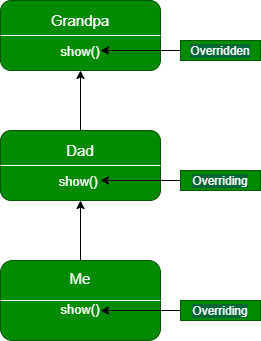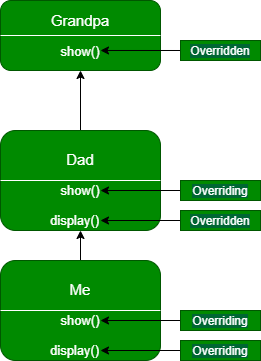Open In App

# Java Program to Use Method Overriding in Inheritance for Subclasses

Method overriding in Java is when a subclass implements a method that is already present inside the superclass. With the help of method overriding we can achieve runtime polymorphism. When we are overriding a method then we must keep three things in mind.

• The method in the subclass must have the same name as the method present inside the superclass.
• The method in the subclass should have the same number of parameters.
• The return type (or sub-type) of the method in the subclass should be the same as the return type of the method inside the superclass.

In this article, we are going to discuss how we can use method overriding in the Inheritance of subclasses.

Let us understand the overriding of the methods of subclasses with the figure given below.In the above figure, we have taken the example of the Grandpa class containing only one method i.e. show(). Dad class inherits the Grandpa class and overrides the show() method. After that Dad class is inherited by Me class which overrides the show() method of Dad class. After that, if we want to access the show() method of the above classes then we can make the object of that class since the method which would be invoked solely depends upon the type of the object but not on the type of reference variable referring to that object.

Let us understand the above concept using code.

## Java

 `// Java Program to show the overriding using inheritance in``// subclasses`` ` `// Grandpa class is at the top of``// the inheritance hierarchy``class` `Grandpa {``    ``public` `void` `show()``    ``{``        ``System.out.println(``            ``"Inside show() method of Grandpa class"``);``    ``}``}`` ` `class` `Dad ``extends` `Grandpa {``   ` `    ``// Overriding show method of Grandpa class``    ``@Override` `public` `void` `show()``    ``{``        ``System.out.println(``            ``"Inside show() method of Dad class"``);``    ``}``}`` ` `// class Me is inheriting Dad class (i.e.``// a subclass of Grandpa class)``class` `Me ``extends` `Dad {``   ` `    ``// Overriding show method of Dad class``    ``@Override` `public` `void` `show()``    ``{``        ``System.out.println(``            ``"Inside show() method of Me class"``);``    ``}``}`` ` `public` `class` `GFG {``    ``public` `static` `void` `main(String[] args)``    ``{``        ``// Creating instance of Grandpa class``        ``Grandpa grandpa = ``new` `Grandpa();``       ` `        ``// Creating instance of Dad class``        ``Grandpa dad = ``new` `Dad();``       ` `        ``// Creating instance of Me class``        ``Grandpa me = ``new` `Me();``       ` `        ``// as discussed which show() function will get``        ``// execute depends upon the type of object`` ` `        ``// show function of Grandpa class will get execute``        ``grandpa.show();``       ` `        ``// show function of Dad class will get execute``        ``dad.show();``       ` `        ``// show function of Me class will get execute``        ``me.show();``    ``}``}`

Output

```Inside show() method of Grandpa class
Inside show() method of Dad class
Inside show() method of Me class```

What happens if a subclass contains methods other than that of the parent/ superclass?

Let us understand this with the help of the figure mentioned below.In the figure above we have taken one more method named display() inside the Dad class which is being overridden inside the Me class. Now if we try to access the display() method by making objects of Dad and Me class respectively and reference variable of Grandpa class, then it will give an error because a reference variable of that class can only be used to access the methods of subclasses that have been overridden from it down the hierarchy, ie we can’t use here reference variable of Grandpa class while calling display function, since Grandpa class is topmost in the hierarchy and it does not contain display function.

We will get the below error if we try to invoke the display() function using the reference variable of Grandpa() class.

```dad.display();
me.display();

GFG.java:52: error: cannot find symbol
^
symbol:   method display()
location: variable dad of type Grandpa
GFG.java:53: error: cannot find symbol
me.display();
^
symbol:   method display()
location: variable me of type Grandpa```

We can solve this problem by making our reference variable of Dad type or Me type refer to the object of Dad and Me class using the syntax mentioned below.

```Dad dad1 = new Dad();
me1.display();```

Now, let us understand these concepts with the help of the code mentioned below.

## Java

 `// Java Program to show the overriding using inheritance in``// subclasses when subclass contains methods other than that``// of the parent/ superclass`` ` `// Grandpa class is at the top of``// the inheritance hierarchy``class` `Grandpa {``    ``public` `void` `show()``    ``{``        ``System.out.println(``            ``"Inside show() method of Grandpa class"``);``    ``}``}`` ` `class` `Dad ``extends` `Grandpa {`` ` `    ``// Overriding show method of Grandpa class``    ``@Override` `public` `void` `show()``    ``{``        ``System.out.println(``            ``"Inside show() method of Dad class"``);``    ``}`` ` `    ``// Creating a display method() for Dad class``    ``public` `void` `display()``    ``{``        ``System.out.println(``            ``"Inside display() method of class B"``);``    ``}``}`` ` `// class Me is inheriting Dad class (i.e.``// a subclass of Grandpa class)``class` `Me ``extends` `Dad {`` ` `    ``// Overriding show method of Dad class``    ``@Override` `public` `void` `show()``    ``{``        ``System.out.println(``            ``"Inside show() method of Me class"``);``    ``}`` ` `    ``@Override` `public` `void` `display()``    ``{``        ``System.out.println(``            ``"Inside display() method of class C"``);``    ``}``}`` ` `public` `class` `GFG {``    ``public` `static` `void` `main(String[] args)``    ``{`` ` `        ``// The code below works the same as in``        ``// previous example``        ``Grandpa grandpa = ``new` `Grandpa();``        ``Grandpa dad = ``new` `Dad();``        ``Grandpa me = ``new` `Me();`` ` `        ``grandpa.show();``        ``dad.show();``        ``me.show();`` ` `        ``// Making Dad type point to the``        ``// Dad object``        ``Dad dad1 = ``new` `Dad();`` ` `        ``// Making Dad type reference variable point to the``        ``// Me object``        ``Dad me1 = ``new` `Me();`` ` `        ``dad1.display();``        ``me1.display();``    ``}``}`

Output

```Inside show() method of Grandpa class
Inside show() method of Dad class
Inside show() method of Me class
Inside display() method of class B
Inside display() method of class C```